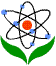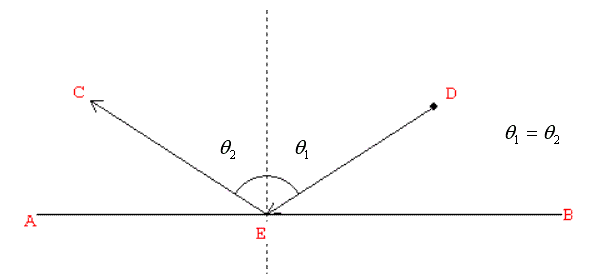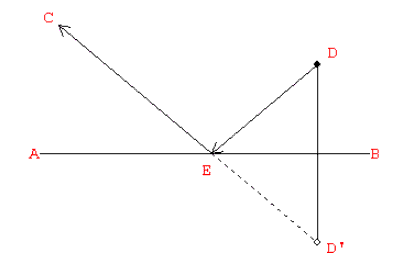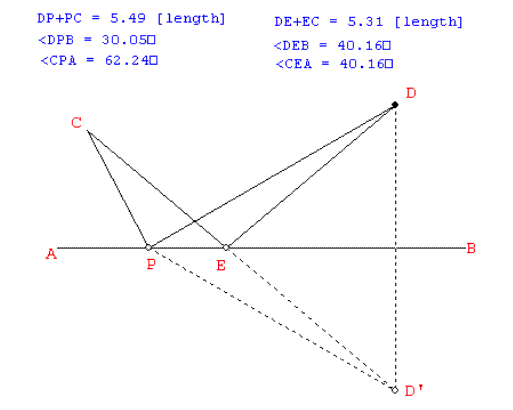Asia-Pacific Forum on Science Learning and Teaching, Volume 5, Issue 3, Article 7 (Dec., 2004) MAN Yiu Kwong Reflection: its concepts and applications in geometry

Reflection and it related concepts in optics

According to Kay (2001), the definition of reflection in a plane is as follows:

Definition: If a transformation f has the property that some fixed line l is the perpendicular bisector of the segment PP' for any point P in the plane and P' = f(P), then f is a reflection with respect to l. The line l is called the line of reflection.

The point P' is called the image of reflection of P. The definition implies that P and P' are located at the opposite sides of l and they are equidistant from l. It is a basic property of reflection. A related concept in optics is the law of reflection, which states that the angle of incidence (the angle between the incoming ray and the normal to the reflecting surface) is equal to the angle of reflection (the angle between the outgoing ray and the normal), as illustrated in Figure 1.Figure 1

This phenomenon is due to the Fermat's principle, which states that light passing through a homogeneous medium (such as air) follows the shortest distance, in order to minimize energy (see Gay, D., 1998 and Hecht, E., 1998). The mathematical reason behind this law of optics can be explained in Figure 2, where D' denotes the image of reflection of D. Since the ray of light takes a path so as to minimize the total distance of travel DE+EC (= D'E+EC), therefore D', E and C must be collinear. Hence, we have CEA =D'EB =DEB and so θ1 = 90o - DEB = 90o - CEA = θ2.Figure 2

Using a dynamic geometry software, such as WinGeom or Sketchpad, this result can be further exemplified or explored by adding an arbitrary point P on AB and then measure the total distance traveled by the light ray from A to E. By dragging the point P along AB, we can see that DP+PC (= D'P+PC) is always greater than DE+EC (= D'E+EC = D'C), provided P and E are distinct points, as shown in Figure 3. In fact, a careful study of the diagram reveals that it is simply a basic result in Euclidean Geometry, which states that the total length of any two sides of a triangle is always greater than the length of the third side.Figure 3